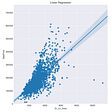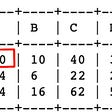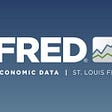# Yield Curve Analytics with Python

We come across the term benchmark yields that is quoted in every financial daily on a regular basis. What exactly does this term mean?. Benchmark yield is the bond yield that is quoted by the market on the sovereign/government bonds trading in that market. It’s called as the benchmark owing to the fact that this is for a sovereign bond with specific maturity (generally 10Y). This yield is like a proxy yield that is used by investors to get a sense of the interest rates in the economy. Investors closely track this yield as it helps them to decide on taking an investment action. Now, just like 10Y yield, there are sovereign securities with other maturities that are traded in the market. For instance, there may be sovereign securities for tenors like 90 days, 180 days, 1 year, 2 year and so on. Generally, securities below 1Y maturity are termed as treasury notes, whereas those with maturity above 1Y are termed as treasury bonds. The yields quoted on such traded bonds are called the YTM i.e. yield-to-maturity. YTM as a yield measure has a few shortcomings which calls for the use of a few analytical techniques to extract additional information that may be used for various financial applications including pricing, modelling etc. In the first part of this post we will discuss an analytical technique widely used by financial market participants. In the subsequent part of this post we will discuss how this technique is implemented in Python.

The technique we will discuss here is deriving the Zero coupon rates (also referred as “ZC” or “Spot Rate” in this post) from the traded market data (i.e. traded yields). Implied forward rate is a related concept to ZC which will also be covered in this post. There are two popular ways to build a ZC curve namely:

Parametric Model: Let’s explore a parametric model for arriving at ZC and implied forward rates. Parametric models are quite elegant in defining the functional form of implied forward rates for each of the required tenors. Subsequently the implied forward rates can be integrated across a continuum of various maturities to arrive at the required ZC for each tenor point.

Functional form of implied forward rates is governed by a set of parameters. These parameters need to be optimized by using a mathematical scheme either like an Ordinary Least Squares or Maximum Likelihood Estimate. Once parameters are optimized to fit the data, these can be used to build the implied forward rates and zero coupon rate curves.

Bootstrapping is another analytical technique wherein we start with the yields with shortest maturity bonds and recursively execute the bootstrapping engine to derive the ZC for further maturity points. This technique will be explored in another post.

In this post the Python libraries that have been used have followed the methodology of Ordinary Least Squares for model parameters fitment.

We will discuss the following models in this post:

· Nelson Siegel model

· Nelson Siegel Svensson model

Let’s discuss briefly the Nelson Siegel model first:

The functional form of this model is:

f (t) = β0 + β1 * exp(-t / Ʈ) + β2 * exp(-t / Ʈ) * (-t / Ʈ)

where, f (t): is the implied forward rate

β0, β1, β2 and Ʈ : model parameters that are to be estimated. These are parameters respectively for long term rate, short term rate, slope and degree of hump.

Integrating the implied forward rates across continuum of maturities yields the zero-coupon rate for a certain tenor given as:

r(t) = β0 + (β1 + β2 ) * (1- exp-(t / Ʈ))/( t / Ʈ) — β2 * exp(-t / Ʈ))

where r(t): zero coupon rate,

t: tenor maturity

Nelson Siegel model is widely used in the financial market for fixed income products. However, there is one drawback i.e. it is unable to handle change in slope and curvature in curve along the tenors. Owing to this an enhancement was suggested via a new model. This model is an extension to the existing Nelson Siegel model called the Nelson Siegel Svensson model.

Let’s discuss briefly the Nelson Siegel Svensson model:

The functional form for of the implied forward rate is similar to that of the Nelson Siegel model however with two additional terms.

f (t) = β0 + β1 * exp(-t / Ʈ1) + β2 * exp(-t / Ʈ1) * (-t / Ʈ1) + β3 * exp(-t / Ʈ2) * (-t / Ʈ2)

and the resulting zero coupon rates would be given by:

r(t) = β0 + (β1 + β2 ) * (1- exp-(t / Ʈ1))/( t / Ʈ1) — β2 * exp(-t / Ʈ1)) + β3 * (1- exp-(t / Ʈ2))/( t / Ʈ2) — β3 * exp(-t / Ʈ2))

where earlier defined terms remain as they are, additionally, Ʈ2 and β3 explain the hump and level and direction of the hump in the curve.

Next, we will see the implementation code for solving the above tedious looking equations. We will leverage powerful quantitative libraries in Python to implement the same with ease.

· Call the libraries that we will be using for developing this model

import numpy as np
import matplotlib.pyplot as plt
from nelson_siegel_svensson import NelsonSiegelSvenssonCurve, NelsonSiegelCurve
from nelson_siegel_svensson.calibrate import calibrate_ns_ols, calibrate_nss_ols

· Next, we input an array of hypothetical market data

#tenors
t = np.array([0.0,0.5,0.75,1.0,1.5,2.0,3.0,4.0,5.0,6.0,7.0,8.0,10.0])

#market rates
y=np.array([0.01,0.02,0.025,0.029,0.03,0.033,0.036,0.039,0.04,0.043,0.045,0.047,0.049])

· Next, we attempt to calibrate the curve i.e. arrive at the various model parameters. We will do it for both the models stated above

curve_fit1, status1 = calibrate_ns_ols(t,y) #NS model calibrate
curve_fit, status = calibrate_nss_ols(t,y) #NSS model calibrate

· Next, we use the parameters arrived at using step above to build the zero coupon and implied forward curve

NS_ZC = NelsonSiegelCurve.zero(curve_fit1,t)
NS_Fwd = NelsonSiegelCurve.forward(curve_fit1,t)

NSS_ZC = NelsonSiegelSvenssonCurve.zero(curve_fit,t)
NSS_Fwd = NelsonSiegelSvenssonCurve.forward(curve_fit,t)

The variables NS_ZC and NS_Fwd will give the zero coupon rates and implied forward rates using Nelson Siegel model, similarly the next two lines of code with variables NSS_ZC and NSS_Fwd will give the output using Nelson Siegel Svensson model.

This is a faster way of building the curve by leveraging Python. A similar implementation can be done by using Excel or pure Python too, however, it may take a longer development time to build these models using the aforesaid tools. Whereas, for rapid prototyping, it’s worth exploring the rich set of libraries that Python has to offer, thereby quickly meeting our end purpose of implementing the model with ease in a few lines of code which also makes the code readable.

--

--

--

## More from Ameya Abhyankar

Founder: FinQuest Institute | Ekspert Consulting; www.finquestinstitute.com; www.ekspertconsulting.com

Love podcasts or audiobooks? Learn on the go with our new app.

## Building a COVID-19 Project Recommendation System## Association mining — Leverage in Python## Real Estate Data## Using Machine Learning to Distinguish Between What’s Real and What Is Not## Statistics and Machine Learning — When to Use What?## Two-sample chi-square test in Python## Method of Initial Rates## Google’s FNet: Mixing Tokens with Fourier Transforms## Ameya Abhyankar

Founder: FinQuest Institute | Ekspert Consulting; www.finquestinstitute.com; www.ekspertconsulting.com

## Stock Analysis using Python (TSA).## Retrieving historical data from FRED or Yahoo Finance using Python## Stock Market Analysis and Prediction using LSTM## FRED API: Get US Economic Data using Python